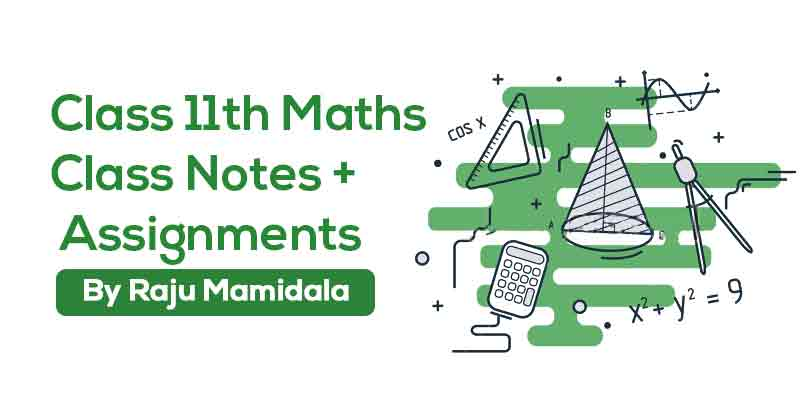## by Raju Mamidala

103 Users Enrolled
Class 11 , Maths
Board: CBSE & State Boards , Language: English

Mode- Online
Contains- 41 PDFS
Access Duration- Till 30 Apr 2019

## WHAT WILL I LEARN?

We aim to prepare this class  students with a complete study material so that the students can score better in the school exam.

Simple and easy to understand.

Understand the level of IIT concepts by these quick revision notes.

Student can test themself with assignments which are provided here.

## Course Description

To become clear with your aspiration and knowledge, you need to begin your preparation from this level. so here you can get complete notes and assignments for preparation along with solutions/Answers.

This study material will help you to better understand the topics and  these notes, students will be able to make quick revisions of topics and score good marks in there exams.

## Curriculum

Complex numbers

• Complex numbers

Compound angles

• Compound angles

Continuety

• Continuety

Cross Product of 2 Vectors

• Cross Product of 2 Vectors

Demoivers theorem

• Demoivers theorem

Differentiation

• Differentiation

Differntiation 2

• Differntiation 2

Directions and cosines

• Directions and cosines

Dot product of 2 vectors

• Dot product of 2 vectors

Errors and Approximation

• Errors and Approximation

Functions

• Functions

Heights and Distances

• Heights and Distances

Hyperbolic functions

• Hyperbolic functions

Inverse Trigonometric functions

• Inverse Trigonometric functions

Limits

• Limits

Locus

• Locus

Logarithms

• Logarithms

Mathematical induction

• Mathematical induction

Maxima and Minima 1

• Maxima and Minima 1

Maxima and Minima 2

• Maxima and Minima 2

Mean value theorem

• Mean value theorem

Multiple and sub multiple

• Multiple and sub multiple

Multiple Product of vectors

• Multiple Product of vectors

Pair of straight lines

• Pair of straight lines

Partial differentiation

• Partial differentiation

Periodicity

• Periodicity

Planes

• Planes

Properties of triangles

• Properties of triangles

Properties of Vectors

• Properties of Vectors

Rate of change

• Rate of change

Real Functions

• Real Functions

Straight lines

• Straight lines

Tangents and normal

• Tangents and normal

Transformations and identities

• Transformations and identities

Trigonometric equations

• Trigonometric equations

Trigonometric expansions

• Trigonometric expansions

Trigonometric ratios

• Trigonometric ratios

## About Raju Mamidala### Raju Mamidala

Mathematics | Class 6th to Competitive exams | 15+ Years Experience | Working in Geethanjali International School in Hyderabad from past 5 years. He Created an Educational Channel for improvement in various aspects for students in IIT Foundation.

#### If you have any doubts about how to get and access this course,watch this video.## 600 399

Includes 18 % GST

Mode- Online

Contains- 41 PDFS

Access Duration- Till 30 Apr 2019